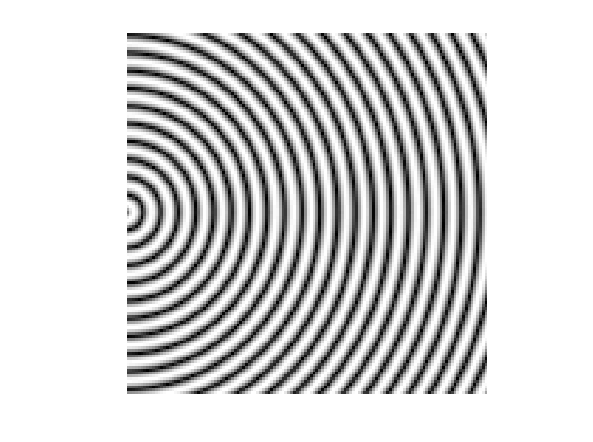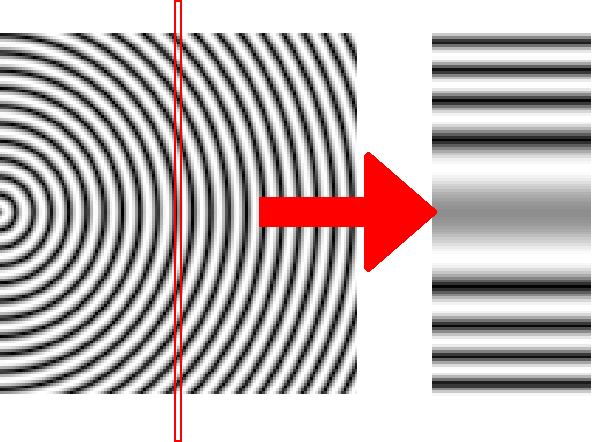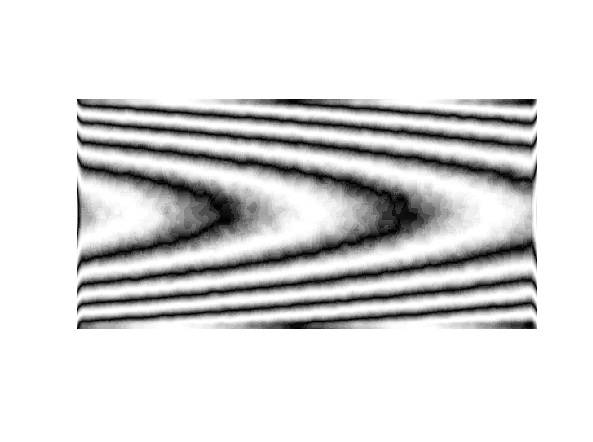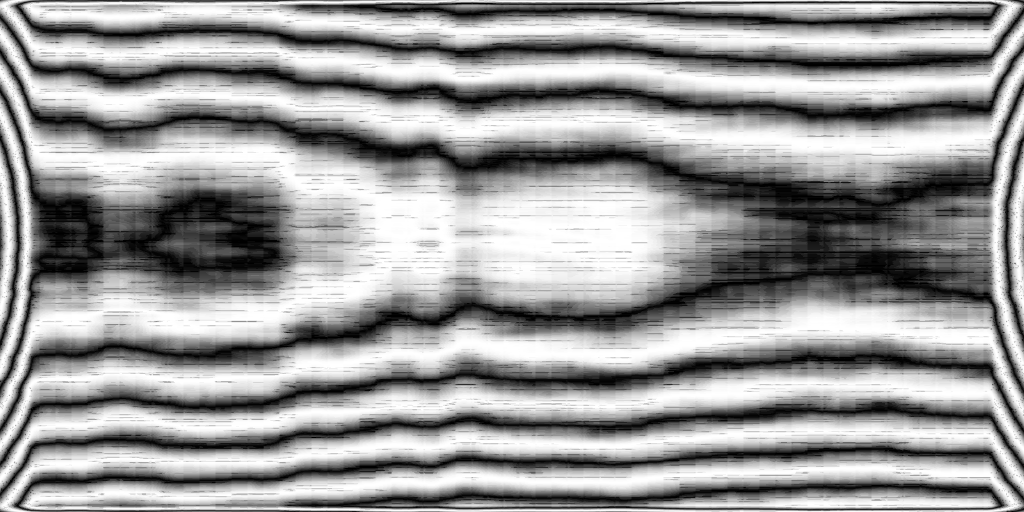# More texture generation (Wood grain)

I started thinking about the trunk of a tree. Essentially, you have a bunch of concentric growth rings. I visualized this as something looking like the image below.The center of the tree trunk would be in the middle of the left side.

Obviously, as you get closer to the top the growth rings shrink and disappear, but I figured it was best to start simple.

This was represented by the following code (GUI Octave)

```for i = 1:y
for j = 1:x
T(i,j) = sqrt((i-(y/2))^2+j^2);
endfor
endfor```
`T=abs(sin(T/s));`

Where x, y, and s were 128, 128, and 8 respectively.

So, if we view this as the trunk, we can “cut” a board out of it by taking a slice.Visualization of “cutting” a board from the trunk of a tree.

This can be achieved with the code

```for i =1:y
for j =1:x
IM(i,j) = T(i,(x/2));
endfor
endfor```

which returns this super boring image

We can also simulate, to some extent, the tree shrinking as we go up the trunk by sloping the “cut”

```for i =1:y
for j =1:x
IM(i,j) = T(i,int8((x/2)+j/(x/4)));
endfor
endfor```

in reality, this is obviously not exactly equivalent to what a perfectly straight tree would look like as the growth rings shrunk, but it is pretty close so we are going to use this for now.

Of course, growth rings are not perfect circles in the real world, but in the same vain as the slope before, we can simulate irregularities by applying an offset.

I randomly generated and smoothed a matrix which I combined with the slice to create the image shown after the code below.

My code, which I admit is not great, is shown below. (The lack of matrix convolution is 100% not because I failed linear algebra and/or I am too lazy to remember)

```x = 128;
y = x;
s = 3;
q=2;
v=8;

R = rand(y,x);
S = zeros(y,x);

for i =q+1:(y-q)
for j =q+1:(x-q)
for k = -q:q
for l = -q:q
S(i,j) = S(i,j)+R(i+k,j+l);
endfor
endfor
endfor
endfor

S=S-((2*q+1)^2)/2;
S=S*s/v;

for i = 1:y
for j = 1:x
T(i,j) = sqrt((i-(y/2))^2+j^2);
endfor
endfor

T=abs(sin(T/s));

for i =2:(y-2)
for j =2:(x-2)
IM(i,j) = T(i,int8(S(i,j)+j/(x/4))+(x/2));
endfor
endfor

imshow(IM)
```

T is the cross cut of the tree, R is a random value matrix, S is a smoothed version of R, and IM is everything combined into the final image shown below.

Or, equivalently, for those that don’t want to waste time, you can use the following code.

```x = 128;
y = x;
s = 3;
q=2;
v=8;

R = rand(y,x);
S = ones(2*q+1,2*q+1);

Z= conv2(R,S,"same");
S=Z-((2*q+1)^2)/2;
S=S*s/v;

for i = 1:y
for j = 1:x
T(i,j) = sqrt((i-(y/2))^2+j^2);
endfor
endfor

T=abs(sin(T/s));

for i =2:(y-2)
for j =2:(x-2)
IM(i,j) = T(i,int8(S(i,j)+j/(x/4))+(x/2));
endfor
endfor

imshow(IM)
```

Granted, this isn’t exactly a high resolution image and we are missing a lot of things including, but not limited to

• knots
• xylem
• branches

but we are moving in the right direction, even if we aren’t doing it in the most efficient manner.

Now, I am going to take a moment to go ahead and sort of bookmark our current code. I made a few modifications to clean it up a bit. I changed from int8 to int16 and bumped up the resolution, as well as changing the aspect ratio.

```T=0;
IM=0;
x = 512;
y = x;
s = 8;
v=.2;
S = fspecial('gaussian',24,9);

R = rand(y,(2*x));

Z= conv2(R,S,"same");
Q=Z-.5;
Q=Q*s/v;

for i = 1:y
for j = 1:x
T(i,j) = sqrt((i-(y/2))^2+j^2);
endfor
endfor

T=abs(sin(T/s));

for i = 1:y
for j = 1:(2*x)
IM(i,j) = T(i,int16(Q(i,j)+j/(x/32))+(x/2));
endfor
endfor

imshow(IM)
```Now, you might notice an interesting effect here. As a byproduct of the gaussian blur we have actually created a bit of a depth map, where the edges of the image look fairly close to what the edges of a board might look like. It isn’t too hard to see how you can generate a UV map for a 3D board that actually matches up on the ends.

There is still a lot more to do, but I am going to stop here for now. Here is a quick preview of the next part.Next time, we add some more variation and a “layer” for the xylem.